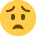Current updates regarding coronavirus (Covid-19) and the precautions AAT are taking will be continually updated on the below page.

We hope you are all safe and well and if you need us we will be here. 💚

# Fin Perf Question Help

Keta operates a standard costing system and uses raw material C2X which is a global community. The standard price was set based upon a market price of £450 per litre when the material price index for C2X was 120.50. The following information has been gathered:
- the price index increased to 126.525 in June X3
- the raw material price variance for June was £375,000 adverse
- 12,500 litres of material C2X were purchased in June

Complete the statements below. In order to calculate your answers, you should split the raw material price variance into two components by calculating the part of the variance explained by the change in the price index and the part of the variance not explained by the changes in the price index.

a) the part of the variance explained by the increase in the price index is £
b) the part of the variance not explained by the increase in the price index is £
c) the percentage increase in the index is %

a) £281,250
b) £93,750
c) 5%

Please helpi have no idea where to start

• Where to start?
We know the material price variance is the difference between the standard cost of the litres of material purchased and the actual amount paid.
1. Here we are told that we bought 12,500 litres and that the standard price per litre was set at £450. This allows us to calculate the standard cost of 12,500 litres
12,500 litres x £450 = £5,625,000
2. We are also told that there was a material price variance which was adverse. This means that when we bought the 12,500 litres we paid more than the standard cost.
Actual cost: £5,625,000 + £375,000 = £6,000,000
3. The index has increased since the standard price was set. We need to revise the standard price to take this into account. Divide by the index value at the time the standard was set and multiply by the index value for the month of the variance.
£450 divided by 120.50 multiplied by 126.525 = £472.50
4. Find the standard cost of the litres purchased using the revised standard price
12,500 litres x £472.50 = £5,906,250
5. We can now separate the £375,000 price variance into the part due to the increase in the price index, and the part due to other factors such as the effectiveness of the purchasing team
6. Original Standard cost £5,625,000
less Revised stdd cost £5,906,250
7. Revised standard cost £5,906,250
Less actual price paid £6,000,000
Price variance (other factors) £93,750 adverse
8. The question of the % increase in the index could be found in point 3. Here just find the difference in the index value (126.525 - 120.50 = 6.025) and divide it by the index value when the standard was set 6.025 divided by 120.50 = 0.05
Multiply by 100 to find the percentage 0.05 x 100 = 5%
This is a link to a book I wrote on management and cost accounting. It has a specific bias towards AAT Financial Performance although it is more general than the main study texts. If you found the above explanation useful you may wish to buy a copy:
http://www.amazon.co.uk/Management-Cost-Accounting-For-Dummies/dp/1118650492

I encourage you to contribute to cancer research UK through the Maureen's Miracles page if you would like to show your appreciation for the answer given:
http://relay.cancerresearchuk.org/site/TR/RelayForLife/General?team_id=6827&pg=team&fr_id=1481

[email protected]
www.sandyhood.com
Sandy
[email protected]
www.sandyhood.com